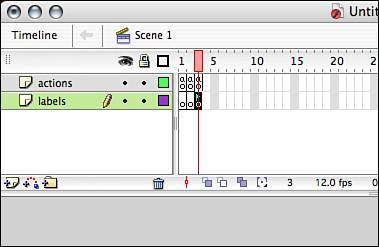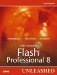# Functions Built In to Flash

We have covered ways of creating and manipulating user-defined functions thus far. Now we'll focus on some of the built-in functions Flash has to offer. We'll briefly go over some basic built-in functions. Finally, we'll discuss some of the deprecated functions.

Because you know what functions look like, I'll only briefly label the parts:

` stop();            //stops a movie clip from playing, and has no parameters play();            //plays a movie clip, and has no parameters gotoAndStop(5);    //goes to a specified frame (the parameter) and stops trace("Flash");    //displays parameter in output window `

This list goes on and on.

However, a couple functions deserve mentioning. One of them is the call function.

#### The call Function

The call function is an interesting function that's brought all the way from Flash 4. This function can run code from any given frame without moving to that frame. It is a deprecated function, and Macromedia recommends using the keyword function to make code available throughout the timeline, as we discussed earlier. However, it's still good to know how to use the call function in case you ever have the need to use it.

The generic template is straightforward. Use the keyword call, followed by a string representing the frame label or a number representing a frame number, enclosed in parentheses. Here is the generic template:

` call(frame); `

Now that you know what it looks like, let's use the call function in an example.

1.

Start a new Flash document.

2.

Then create a new layer. Name the top layer labels and the bottom layer actions. Then place two more keyframes in each layer (F6).

3.

Open the Actions panel for the first frame on the Actions layer and place this code there:

` stop(); `

This will stop the play head from moving past this frame.

4.

Next, in frame 2 of the same layer, place this trace statement:

` trace("This is code from frame two"); `

5.

Then in the final frame of the Actions layer (frame 3), place this TRace statement:

` trace("This is code from the labeled frame"); `

6.

Now we turn our attention to the label's layer. In the third frame of this layer, place the label "labeled." When you're done, your screen should look similar to Figure 12.1.

##### Figure 12.1. The project thus far.7.

If you were to test this movie right now, nothing would happen. This is because of the stop function in frame 1. If stop were removed, both trace statements would run repeatedly, over and over, while the movie loops through. However, when you place these next actions after stop, both statements will be run, but only once, and the frames will never be reached by the play head. So place the following code in the first frame of the Actions layer, and at the end, it will look like this:

` stop(); call(2); call("labeled"); //output: This is code from frame two //        This is code from the labeled frame `

Test the movie. There you have itthe code that was placed in frame 2 as well as the third frame, which was labeled "labeled," runs without the play head ever reaching these frames.

Now that we have covered this unique function, let's move on to some more built-in functions. Flash has predefined a couple of categories for functions in the Actions panel. One such category is the conversion functions.

#### Conversion Functions

Conversion functions perform a specific task on objects: they convert objects. Each of the five main data types has its own conversion script that will change it to another data type.

The generic template utilizes the keyword of the object you are trying to convert to, and the expression you are trying to convert follows, enclosed in parentheses, as shown here:

` converter(expression); `

We will use the following example to change some data from one type to another. After each step, we will use the TRace function and the typeof operator to display the data type of each object following the conversion. Here's the code:

` //Start off with a simple string myString = "string"; trace (typeof myString); //Now we begin converting the same object //again and again while checking after each time myString = Number(myString); trace (typeof myString); myString = Array(myString); trace(typeof myString); myString = Boolean(myString); trace(typeof myString); myString = Object(myString); trace(typeof myString); //Finally back to a string myString = String(myString); trace(typeof myString); //output: string //        number //        object //        boolean //        object //        string `

NOTE

When converting to an array with the Array conversion function, whatever is being converted will be placed in the first element of the array and not separated into individual elements.

Converting data types is an important part of using ActionScript; however, the preceding code will not work with strict data typing, so keep that in mind if you want to change the data type of a variable over and over (which is bad practice). You can convert numbers from input text fields (all types of information from input text fields are strings; see Chapter 15, "Working with Text," for more information) into true number data types. You can convert Boolean data types into strings to use in sentences.

Let's now move on to the next category of functions: mathematical functions.

#### Mathematical Functions

Mathematical functions execute mathematical operations on expressions you assign to them. You may be thinking addition, subtraction, and so on, but these operations are much more advanced. Only four mathematical functions are listed in ActionScript:

NOTE

Although only four mathematical functions are in Flash, there is also the Math object, which has several methods for performing other mathematical calculations.

• isFinite

• isNaN

• parseFloat

• parseInt

The first two of the mathematical functions act more like conditionals. Let's see how they work individually.

The first, isFinite, checks to see whether the expression entered is a finite number. If the number is finite, the function returns true. If the expression is not finite (or infinite), the function returns false. Here is an example in which we test two numbers and trace the results:

` trace(isFinite(15)); //evaluates to true trace(isFinite(Number.NEGATIVE_INFINITY)); //evaluates to false `

The second of these two functions, isNaN, works in the same manner. It checks to see whether the expression entered is not a real number. If the expression is not a real number, the function returns true. If the expression is a real number, the function returns false. Let's take a look:

` trace(isNaN(15)); //evaluates to false trace(isNaN("fifteen")); //evaluates to true trace(isNaN("15")); //evaluates to false `

Even though the last example is in fact a string, the interpreter converted it to a number when it was evaluated. Keep this in mind when evaluating numbers as strings.

The next mathematical function is parseFloat. This function takes numbers out of a string literal until it reaches a string character. Then it converts what it has removed into a true number data type. Here are a few examples:

` trace(parseFloat("15")); //output: 15 trace(parseFloat("fifteen")); //output: NaN trace(parseFloat("20dollars")); //output: 20 `

As you can see in the preceding example, the function takes only the number and drops the rest of the string.

The last mathematical function is parseInt. This function can perform the same task as the parseFloat function, but it can also use a radix, which is useful when working with octal numbers. Here is an example:

` trace(parseInt("15", 8)); //output: 13 (a representation of the octal number 15 //that has been parsed) `

A few more functions are defined directly in ActionScript, including getProperty(), getTimer(), targetPath(), and getVersion(), all of which return information. There is also eval, escape, and unescape, which perform their desired tasks on expressions.

We have covered a great deal of information about functions; now let's look at some deprecated functions and alternatives to their use.

#### Deprecated Functions

If you have worked in Flash 4 or even Flash 5, you may notice that some of the functions are not where they used to be. No, they are not completely gone, but they are deprecated, which means that ActionScript provides new ways of performing the same tasks, and although these functions are still available for use, they might not be in the next release. Therefore, it is a good idea to get out of the habit of using them.

We'll go over each deprecated function briefly and discuss alternatives to their use.

##### chr

The chr function converts a numeric value to a character based on ASCII standards. It has been replaced by String.fromCharCode. Here are examples of both:

` //The old way trace(chr(64)); //The new way trace(String.fromCharCode(64)); //output @ //       @ `

##### int

The int function takes a number with a decimal point and drops the decimal point. It has been replaced by Math.floor. Here are examples of both:

` //the old way trace(int(5.5)); //the new way trace(Math.floor(5.5)); //output: 5 //        5 `

##### length

The length function returns the number of characters in a string or variable holding a string. It has been replaced by String.length. Here are examples of both:

` //First create a variable holding a string myString = "Flash"; //the old way trace(length(myString)); //the new way trace(myString.length); //output: 5 //        5 `

##### mbchr

The mbchr function, like the chr function, converts a numeric value to a character based on ASCII standards. It has been replaced by String.fromCharCode. Here are examples of both:

` //the old way trace(mbchr(64)); //The new way trace(String.fromCharCode(64)); //output @ //       @ `

##### mblength

The mblength function, like the length function, returns the number of characters in a string or variable holding a string. It has been replaced by String.length. Here are examples of both:

` //First create a variable holding a string myString = "Flash"; //the old way trace(mblength(myString)); //the new way trace(myString.length); //output: 5 //        5 `

##### mbord

The mbord function converts a character to a number by using the ASCII standard. It has been replaced by String. CharCodeAt. Here are examples of both:

` //the old way trace(mbord("@")); //the new way trace(("@").charCodeAt(0)); //output: 64 //        64 `

##### mbsubstring

The mbsubstring function removes a set number of characters from a string. It has been replaced by String.substr. Here are examples of both:

` //First, create a variable to hold a string myVar = "Unleashed"; //the old way trace(mbsubstring(myVar, 0, 2)); //the new way trace(myVar.substr(0, 2)); //output: Un //        Un `

##### ord

The ord function, like the mbord function, converts a character to a number by using the ASCII standard. It has been replaced by String. CharCodeAt. Here are examples of both:

` //the old way trace(ord("@")); //the new way trace(("@").charCodeAt(0)); //output: 64 //        64 `

##### random

The random function returns a random number from a expression given. It has been replaced by Math.random. Here are examples of both:

` //the old way trace (random(5)); //the new way trace (Math.floor(Math.random()*5)); //output: (2 random numbers between 0-4) `

##### substring

The substring function, like the mbsubstring function, removes a set number of characters from a string. It has been replaced by String.substr. Here are examples of both:

` //First, create a variable to hold a string myVar = "Unleashed"; //the old way trace(substring(myVar, 0, 2)); //the new way trace(myVar.substr(0, 2)); //output: Un //        Un `

This is the last of the deprecated functions.Macromedia Flash Professional 8 Unleashed
ISBN: 0672327619
EAN: 2147483647
Year: 2005
Pages: 319